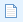Contents - Index

Accounts: Lease - Depreciation

Used to set up and track depreciation for the lease.

ALMSys allows multiple iterations of depreciation for the same asset, meaning that you may stop and start depreciation as many times as you wish. The Start Value on the first iteration is the amount from which depreciation will begin. Subsequent start values are only used to calculate the new monthly book amount.

Start Value is the original cost of this particular asset.

Book Term is the number of months that the asset will be depreciated using the standard book method. (However, book depreciation will continue until explicitly terminated on this screen, or until the Book Value equals zero.)

The start date should be set to the first of the month for the month in which depreciation started. For example, if the lease date is 11/08/2016, and you want to start depreciating in November, then set the depreciation start date to 11/01/2016.

Accum. Depr. Adjustment is only used in the case where you need the accumulated depreciation altered to match your ledger.

End Date is used to terminate this iteration of depreciation. If you are completely terminating depreciation, use the Overall Depreciation End Date field. (It is recommended to set the end date to the last day of the month through which you are depreciating.)

Book Value is, as of today (or as of the "Calculation As Of" date), the current depreciation book value. This is simply Start Value minus Accumulated Depreciation.

Use the Create Journal button to create a journal or reverse journal for the current "Months Into" number.

Use the Copy Values from Lease button to copy lease values into the depreciation calculation.

Use the Copy Values from a Different Deal button to copy depreciation values from any other deal (that has depreciation).

Changing Depreciation
If you have already depreciated one month or more and wish to alter depreciation to come up with a different Monthly Book amount, then please follow these steps.

1. Stop the current iteration by entering an End Date.

2. Click thebutton that is located above the blue Depreciation Iterations grid. It will start a new iteration with values where the prior depreciation left off.

3. Alter the depreciation fields, including the start value, to whatever is needed to come up with the desired Monthly Book amount. Depreciation will continue at the value of where it left off from the prior iteration.